# Market Statistics (Volume Histogram, VWAP with SD bands) - page 29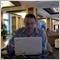Moderator
126161

Elite indicators :)

William Snyder, 2012.01.28 09:54

Maybe this will be of some use for you Simba,or maybe a starting point, the bands are using Volume Ma with jurik(no ticks though), the profile is using ticks selectable by user, the market profile(mp) is basically in the include file and library files, called by the indicator. The mp has 2 modes, mode 1 is using one or all three trading sessions, the mode 2 you can make your own time called by csv file. From what i remember of J-Charts, using mp in text mode seems similar.

Files:Moderator
126161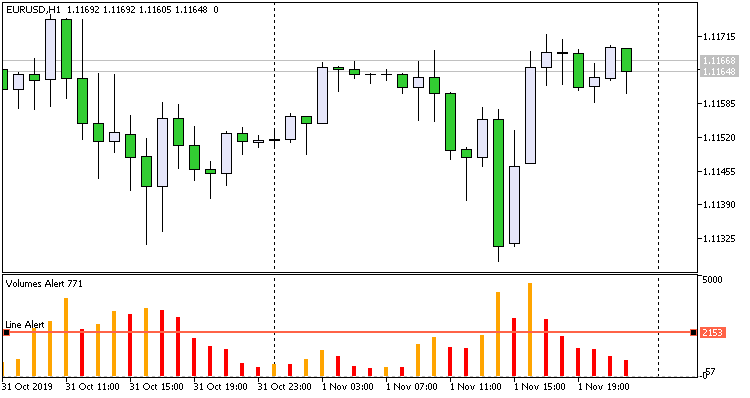Based on the iVolumes indicator: the user puts the “Horizontal line” graphic object (OBJ_HLINE) in the indicator window. When the indicator reaches or crosses this line, a sound will be played and Alert will be issued.

Settings:

• the line name must match the " Line Alert " parameter
• preset sound " Sound Name "
• plays the specified number of times " Repetitions "
• with a given pause " Pause "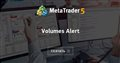• www.mql5.com
На основе индикатора iVolumes: пользователь наносит графический объект (OBJ_HLINE) "Горизонтальная линия" в окно индикатора. Когда индикатор достигнет или пересечёт эту линию, будет проигран...14

Hi!

I am wondering if it is still possible to download version 6_2 and 7 of the Market Statistics indicator?

Thanks137

GormTheOld:

Hi!

I am wondering if it is still possible to download version 6_2 and 7 of the Market Statistics indicator?

Thanks

Here you are!

Files:8

Has anyone seen MIDAS Top/Bottom finder for MT5 or tried to code it based on the TradeStation code as below?

```Input: Vol_D(0), //Input Volume ("D" from Levine’s //formula)
TBF_Price(L), //Bar Price to use; suggest "L" for
//TopFinder and "H" for BottomFinder;
//Alternatively, "(H+L+C)/3"
//may be used for an average price

MyVolume(Ticks), //Bar Volume information to use;
//suggest "Ticks", or "1"
//if no volume information
//"Ticks" yields total volume,
//and "Volume" yields only

StartingDate(1120101), //Start Date in TradeStation date
//format: YYYMMDD, where YYY
//is years since 1900
//Example date is January 1, 2012

StartingTime(1530), //Start Time in military time with no
//punctuation; example time is 3:30 pm

//StartColor and end with EndColor ...
EndColor(Red); //... and changes according to %
//D completion

Vars: running(false), //whether or not TBF calculation has
//started and not ended
pv(0), //cumulative price ∗ volume
vol(0), //cumulative volume
_D(0), //variable that holds input volume, D
pvInt(0), //interpolated pv
j(0), //loop iterator
e(0), //same as Levine’s "e" variable
eT(0), //temporary copy of "e" used for
//iteration
tbf(0), //current calculated price of TBF curve
pct_D(0); //percent completion of TBF curve
//begin at user specified date and time
if (date = StartingDate and time = StartingTime) or running then begin
running = true;
pv = pv + TBF_Price ∗ MyVolume; //add current bar’s price ∗
//volume to cumulative total
vol = vol + (MyVolume); //running total of volume
//begin calculation of TBF price
if Vol_D <> 0 then begin
_D = Vol_D; //store copy of input volume
e = vol ∗ (1 - vol / _D); //calculate "e" per
//Levine’s formula
//if "e" greater than zero, continue to
//calculate TBF price
//otherwise, TBF is completed
if e > 0 then begin
eT = e; //temporary
//copy of "e"
j = -1; //used for iteration
//iterate backwards until the cumulative
//displaced volume is greater than or
//equal to "e"
while eT > 0 begin
j = j + 1;
eT = eT - MyVolume[j];
end;
//If displaced volume is greater than "e"
//(nearly always),
//an interpolated pv amount is calculated
//for "j" bars ago using only that part of
//"j" bar’s volume needed to make cumulative
//displaced volume equal to "e".
//Note that at this point, "eT" is negative
//and contains the partial volume
//of "j" bars ago that should be excluded.
if eT < 0 then pvInt = TBF_Price[j] ∗
(MyVolume[j] + eT) else pvInt = 0;
tbf = (pv - pv[j] + pvInt) / e; //calculate
//TBF curve
//price for
//this bar
pct_D = vol / _D ∗ 100; //calculate
//percent TBF
//completion
plot1(tbf, "TBF");
//Set Plot Color based on gradient between two
//Input colors
//StartColor, EndColor));
end
else running = false; //TBF curve is completed; do
//not run anymore
end;
end;
```5

interestingModerator
126161

Vsa learning club

Sergey Golubev, 2022.01.20 08:37

Volume Extremes - indicator for MetaTrader 5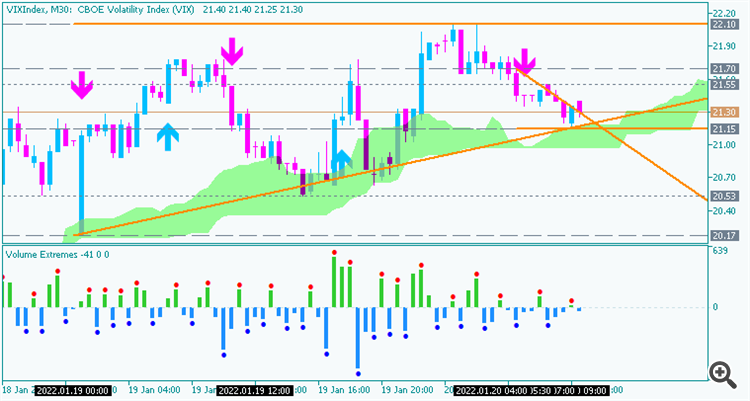iVolumes (Volumes) indicator in the form of a colored histogram. Bar drawing rule: if the bar is bullish, then the bar is drawn above zero, if the bar is bearish, it is drawn below zero. Additionally, a search is made for extremums on the indicator - three columns are compared

We can use this indicator for trading to place the levels (please note that the levels are dynamically changed so it is necessary to re-place the levels in periodical way), for example: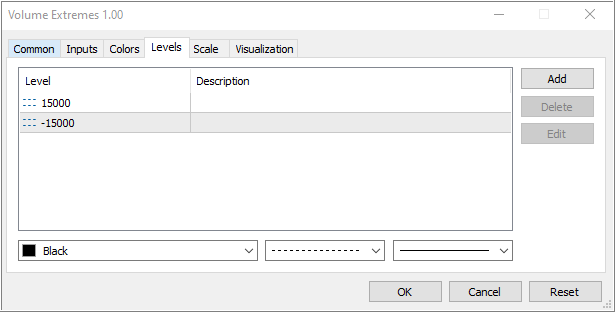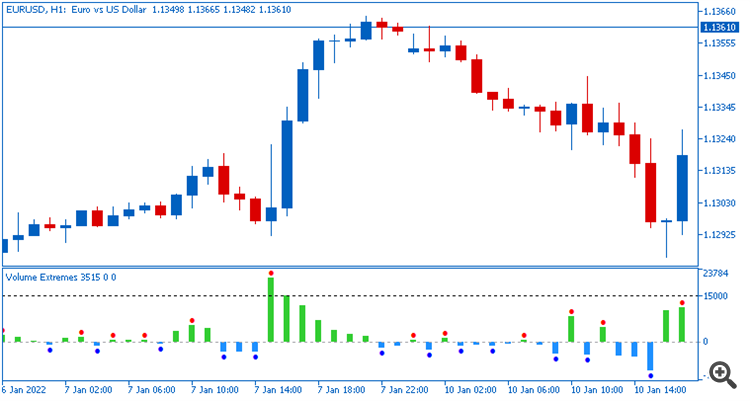Moderator
126161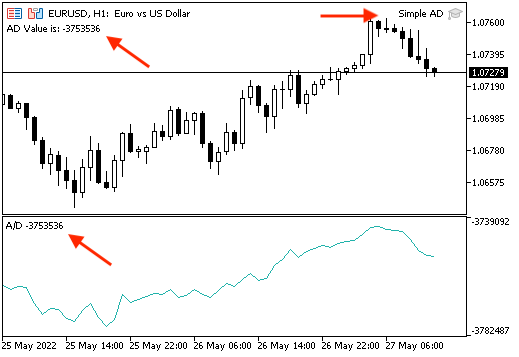In this new article from our series is about learning about the most popular technical indicators in detail by learning how we can use them by simple strategies. Then designing trading systems for these strategies by MQL5 to execute these trading systems in the MetaTrader 5 trading platform, we will learn about a new technical indicator that is one of the volume family indicators that can show us another perspective about the instrument which is the volume measurement, this indicator is the Accumulation/Distribution which is called (AD) also. We will cover this indicator through the following topics: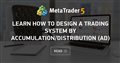• www.mql5.com
Welcome to a new article from our series about learning how to design trading systems based on the most popular technical indicators, we will learn in this article about a new technical indicator that is Accumulation/Distribution indicator, and how to design a trading system by MQL5 based on simple AD trading strategies to be able to use them in the MetaTrader 5.Moderator
126161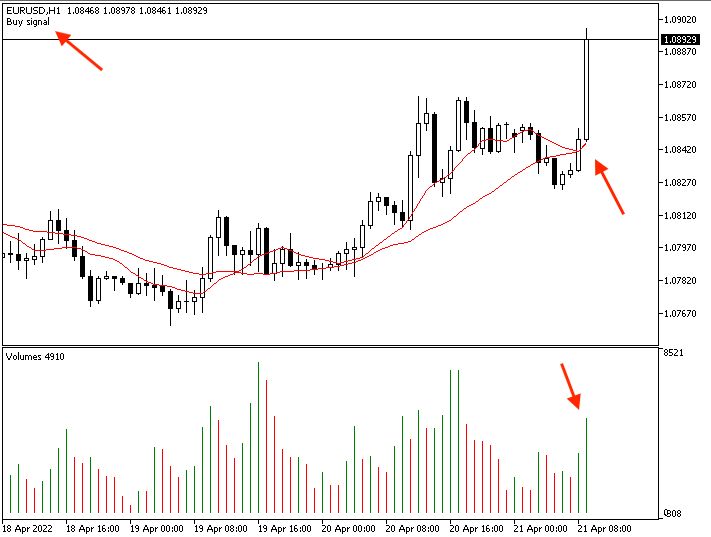Welcome to this new article in our series about learning how to design a trading system based on the most popular technical indicator. Here is a new important technical indicator that gives us insights about a different perspective in the market - the Volumes indicator. We will cover this indicator through the following topics: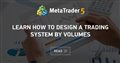Learn how to design a trading system by Volumes
• www.mql5.com
Here is a new article from our series about learning how to design a trading system based on the most popular technical indicators. The current article will be devoted to the Volumes indicator. Volume as a concept is one of the very important factors in financial markets trading and we have to pay attention to it. Through this article, we will learn how to design a simple trading system by Volumes indicator.